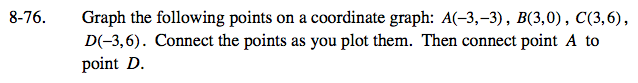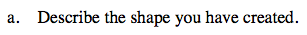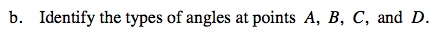### Home > CC2 > Chapter 8 > Lesson 8.3.2 > Problem8-76

8-76.
1. Graph the following points on a coordinate graph: A(−3, −3), B(3, 0), C(3, 6), D(−3, 6). Connect the points as you plot them. Then connect point A to point D. 8-76 HW eTool (Desmos). Homework Help ✎

1. Describe the shape you have created.

2. Identify the types of angles at points A, B, C, and D.There is only one pair of parallel sides.Notice that lines BC and AD are perpendicular to line CD.

Use the eTool below to plot the points.
Click the link at right for the full version of the eTool: CC2 8-76 HW eTool